## Determinants

#### Introduction

The determinant of a 2x2 matrix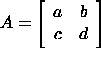is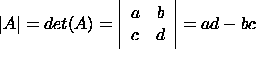Notice the difference in notation between the matrix and its determinant: matrices are typically enclosed with square brackets whereas determinants of matrices are enclosed by straight lines. The determinant is a scalar quantity. It contains much information about the matrix it came from and is quite useful in many applications. The determinant is most often used to

#### Example

The determinant of the matrix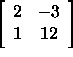is given by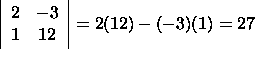#### Determinants of Larger Matrices

The determinant of a 3x3 matrix is a bit more involved:which, after taking the three 2x2 determinants, is equivalent to |B|=a(ek-fh)-b(dk-fg)+c(dh-eg). This is called the expansion of the determinant by its first row. To do this cross out the row and column containing the first element of the first row (the number a). We are left with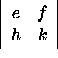Now take this determinant and multiply it by a (the element that was crossed out). Repeat the process for the second and third elements of the first row. These terms are then combined with alternating signs, +, -, +. The result is the quantity shown above. The determinant of any nxn matrix can be found by expanding in this manner on any row or column.

#### Example

The determinant of the matrix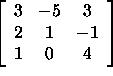is given by#### An Alternative Computational Approach

The determinant of a 3x3 matrix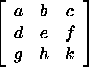can also be found using the following method: repeat the first two columns of the matrix to the right of the last column: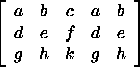Now, the determinant is the sum of the products of the upper left to lower right diagonals minus the sum of the product of the upper right to lower left diagonals: |A|=(aek+bfg+cdh)-(bdk+afh+ceg). Compare this result with the one obtained above.

Copyright © 1996 Department of Mathematics, Oregon State University

If you have questions or comments, don't hestitate to contact us.